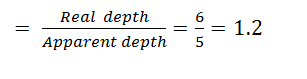# One face of a rectangular glass plate 6 cm thick is silvered. An object held 8 cm in front of the first face forms an image 12 cm behind the silvered face. The refractive index of the glass is (a)0.4 (b) 0.8 (c)1.2 (d)1.6Navjyot Kalra
10 years ago

(c)

Thickness of glass plate (t) = 6 cm;

Distance of the object (u) = 8 cm.

And distance of the image (v) = 12 cm.

Let x = Apparent position of the silvered surface in cm,

Since the image is formed due to reflection at the silvered face and by the property of mirror image

Distance of object from the mirror = Distance of image from the mirror

or, x + 8 = 12 + 6 – x Þ x = 5 cm.

Therefore, refractive index of glass5 years ago
The distance of object form silvered surface= 8+6= 14cm The distance of image from silvered surface = 12 cmRefractive index = The distance of object form silvered surface/ The distance of image from silvered surfaceRefractive index = 14/12 = 1.1667 = 1.2Kushagra Madhukar
3 years ago
Dear student,

Thickness of glass plate (t) = 6 cm;
Distance of the object (u) = 8 cm.
And distance of the image (v) = 12 cm.
Let x = Apparent position of the silvered surface in cm,
Since the image is formed due to reflection at the silvered face and by the property of mirror image
Distance of object from the mirror = Distance of image from the mirror
or, x + 8 = 12 + 6 – x
Hence, x = 5 cm.
Therefore, refractive index of glass = real depth/apparent depth = 6/5 = 1.2

Hope it helps.
Thanks and regards,
Kushagra